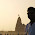### Original Puzzle: Pattern Lock - Combinatorics Puzzle - Number of Possible Passwords

Source: Discussion with Ankush Jain (CSE IITB 2011 Alumnus, Morgan Stanley Analyst) a few months back. Discussion revived by Sangram Raje (CSE IITB 2008 Alumnus, Tower Research Analyst) today.

Problem:
Ever seen a pattern lock in Galaxy S2? Password is a series of connected line strokes. How many possible password combinations can you have?

1) Assuming the dots on the screen are like (1, 2, 3 in the first row), (4, 5, 6 in the second row) and (7, 8, 9 in the third row), you cannot go to 8 from 2, without going through 5. So, a password like * * 8 2 * * is not possible.
2) You cannot move over two lines twice You can move to a used point, but you cannot move to another used point from a used point

I do not see a simple way to solve this. But even coding this looks very difficult to me. Any takers?

Update: (19-07-2012)
This is essentially an open ended question

1.Coding at least is easy. Answer comes to 140240. I have assumed that a single number is not a valid password, and otherwise there is no restriction that all numbers must be used.

2.Coding does not seem so hard. Even a brute force approach seems to finish in a few minutes to give 149507072.

Code as follows, let me know if this looks okay:

#include

#define N 3
#define NN 9

void countPasswords (int* pickedArr, bool lastAlready, int lastPicked, int depth, int& count, int nextArr[][NN]) {
if (depth >= (NN + 1)) {
// return;
}

for (int i = 0; i < NN; i++) {
if ((lastPicked >= 0) && (nextArr[lastPicked][i] == 0)) {
continue;
}

bool lastThis = (pickedArr[i] > 0);

if (lastAlready == true && lastThis == true) {
continue;
}

++ pickedArr[i];

if (depth > 0) {
++ count;

// for (int k = 0; k < NN; k ++) {
// printf ("%d ", pickedArr[k]);
// }

// printf ("\n");
}

countPasswords (pickedArr, lastThis, i, depth + 1, count, nextArr);
-- pickedArr[i];
}

}

int main (void) {
int pickedArr[NN];

for (int i = 0; i < NN; i ++) {
pickedArr[i] = 0;
}

int lastPicked = -1;
int count = 0;
int nextArr[NN][NN];

for (int i = 0; i < NN; i++) {
int i1 = (i / N);
int i2 = (i % N);

for (int j = 0; j < NN; j ++) {
int j1 = (j / N);
int j2 = (j % N);

if (((i1 + j1) % 2 == 0) && ((i2 + j2) % 2 == 0)) {
nextArr[i][j] = 0;
} else {
nextArr[i][j] = 1;
}

// printf ("%d ", nextArr[i][j]);
}

// printf ("\n");
// printf ("\n");
}

int depth = 0;
countPasswords (pickedArr, false, lastPicked, depth, count, nextArr);
printf ("count %ld\n", count);
}

3.The solution is possible only on theoretical basis as per my knowledge.
Because practically,the time lapse between two WRONG hits,increases exponentially.

4.I have an approach..please validate :)

First lets define three functions:-
T(k) = No of passwords using k vertices
F(k) = No of passwords where starting point = end point
G(k) = no of passwords where starting point is different from ending point

Argument 1:- T(k) = F(k) + G(k)
ie. All possible paths = Paths that start from a point and don't end at that point + Paths that start from a point and end at that point

Argument 2:- T(k+1) = (k+1)*(2.T(k)+k-k.F(k))
ie. If we add a vertex to our set of k vertices, the total number of paths =
Number of paths with edge > 1, that start from the new vertex but don't end in the same vertex = T(k) + paths that start and end on the same new vertex = T(k)
Plus number of paths with edge length one = k
Minus number of paths that start with some other vertex and end at the same vertex = k.(F(k))
For k+1 vertices...by symetry...All paths will be unique because we are defining unique starting vertices.

Now we need to find F(k)
Its actually easier to find G(k), so lets do that:-

Argument 3:- G(k+1)= (2.G(k)+k)(K+1)
No of paths which start with new vertex and does not end with the same vertex = (2.G(k) + k) why?...think

Solving the recurrence relation for G(k) and replacing F(k) by T(k)-G(k) in argument 2 and solving that recurrence relation should give the answer.

I am rusty with solving rec rel's...but comments are welcome...

Jatin Patni

5.@Pyrole, your solution assumes that it is possible to move to any vertex from any vertex, which is not the case here
like for example, we cannot move to 9 from 1 directly. So, we need to make a graph and then try to find the solution(which perhaps can be done by a little modification to DFS)